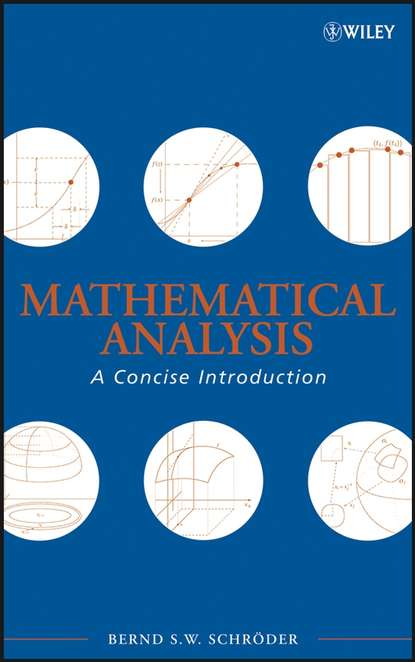Последний отзыв
Аноним
09.03.2023
Супер, на одном дыханииОценить:Рейтинг: 0

# Mathematical Analysis

Жанр
Год написания книги
2019
A self-contained introduction to the fundamentals of mathematical analysis Mathematical Analysis: A Concise Introduction presents the foundations of analysis and illustrates its role in mathematics. By focusing on the essentials, reinforcing learning through exercises, and featuring a unique «learn by doing» approach, the book develops the reader's proof writing skills and establishes fundamental comprehension of analysis that is essential for further exploration of pure and applied mathematics. This book is directly applicable to areas such as differential equations, probability theory, numerical analysis, differential geometry, and functional analysis. Mathematical Analysis is composed of three parts: ?Part One presents the analysis of functions of one variable, including sequences, continuity, differentiation, Riemann integration, series, and the Lebesgue integral. A detailed explanation of proof writing is provided with specific attention devoted to standard proof techniques. To facilitate an efficient transition to more abstract settings, the results for single variable functions are proved using methods that translate to metric spaces. ?Part Two explores the more abstract counterparts of the concepts outlined earlier in the text. The reader is introduced to the fundamental spaces of analysis, including Lp spaces, and the book successfully details how appropriate definitions of integration, continuity, and differentiation lead to a powerful and widely applicable foundation for further study of applied mathematics. The interrelation between measure theory, topology, and differentiation is then examined in the proof of the Multidimensional Substitution Formula. Further areas of coverage in this section include manifolds, Stokes' Theorem, Hilbert spaces, the convergence of Fourier series, and Riesz' Representation Theorem. ?Part Three provides an overview of the motivations for analysis as well as its applications in various subjects. A special focus on ordinary and partial differential equations presents some theoretical and practical challenges that exist in these areas. Topical coverage includes Navier-Stokes equations and the finite element method. Mathematical Analysis: A Concise Introduction includes an extensive index and over 900 exercises ranging in level of difficulty, from conceptual questions and adaptations of proofs to proofs with and without hints. These opportunities for reinforcement, along with the overall concise and well-organized treatment of analysis, make this book essential for readers in upper-undergraduate or beginning graduate mathematics courses who would like to build a solid foundation in analysis for further work in all analysis-based branches of mathematics.

## Отзывы

список сообщений пуст